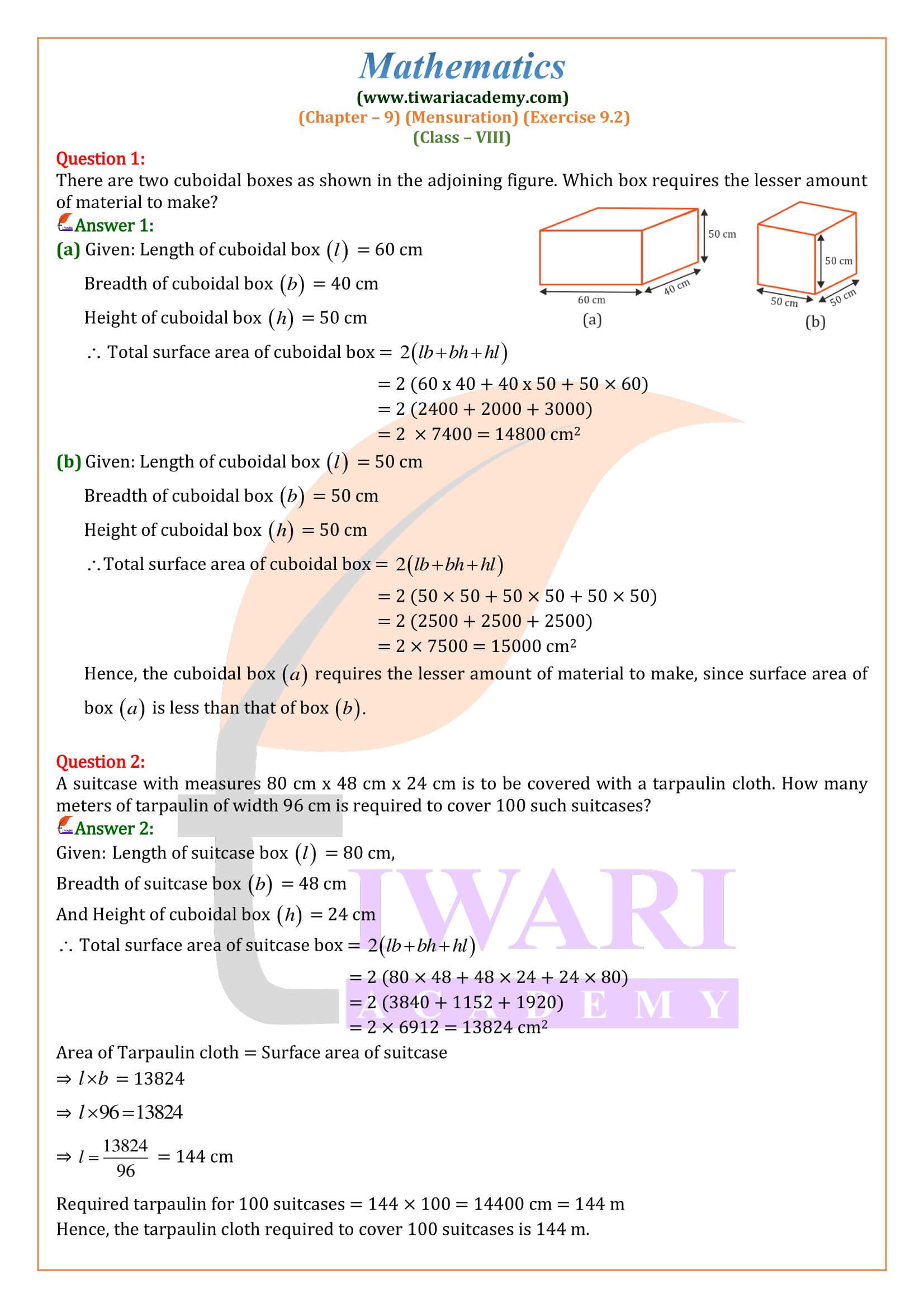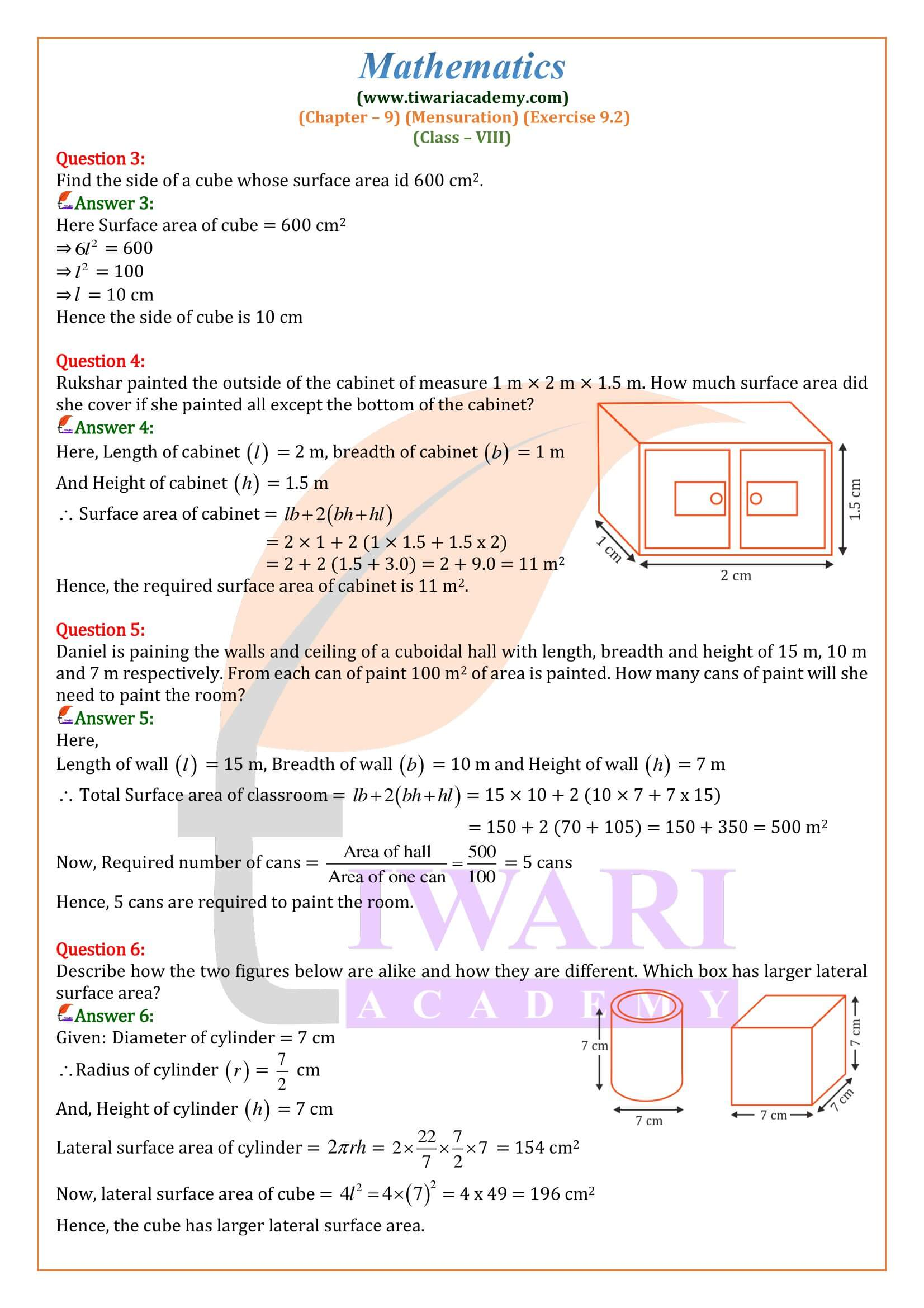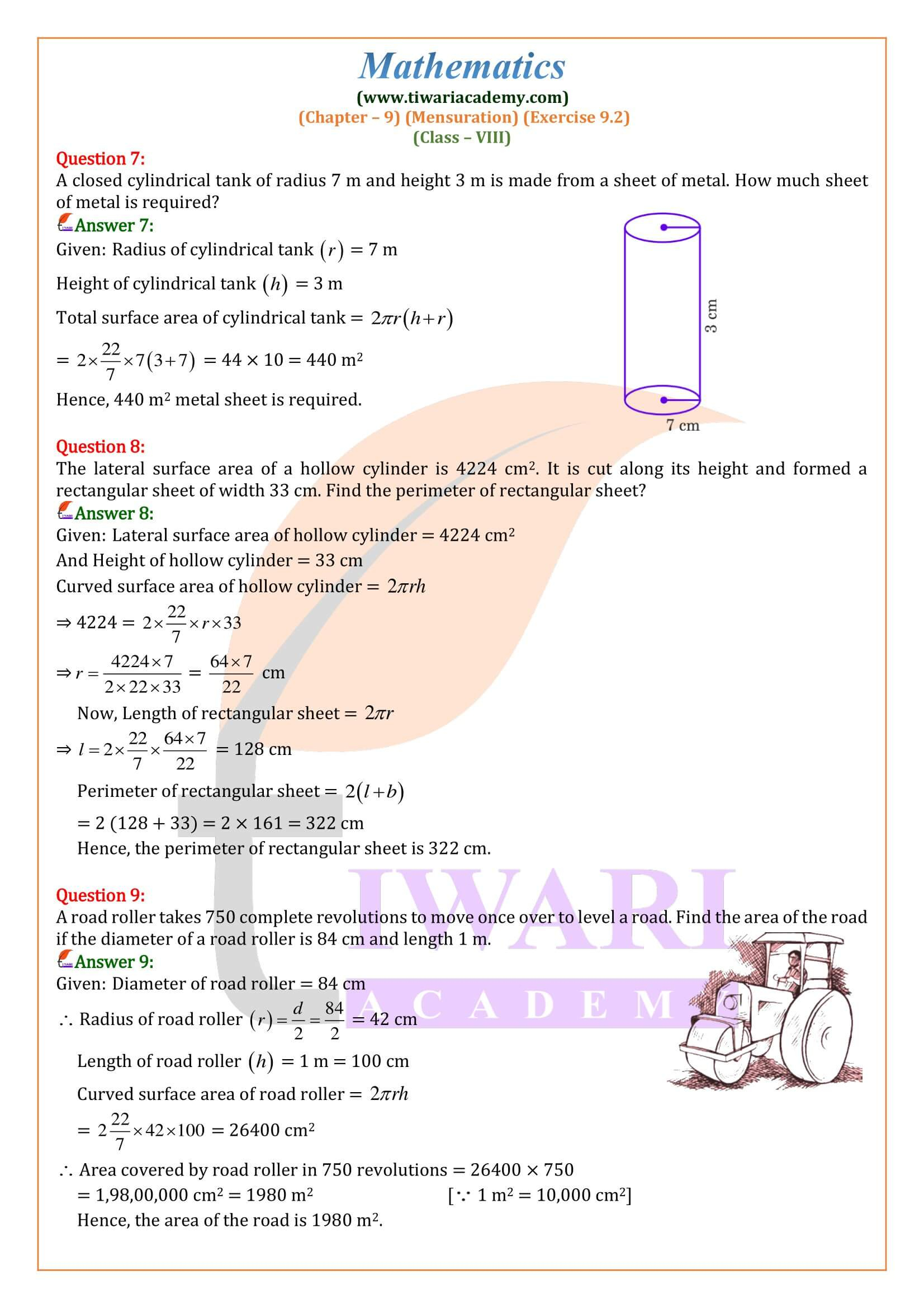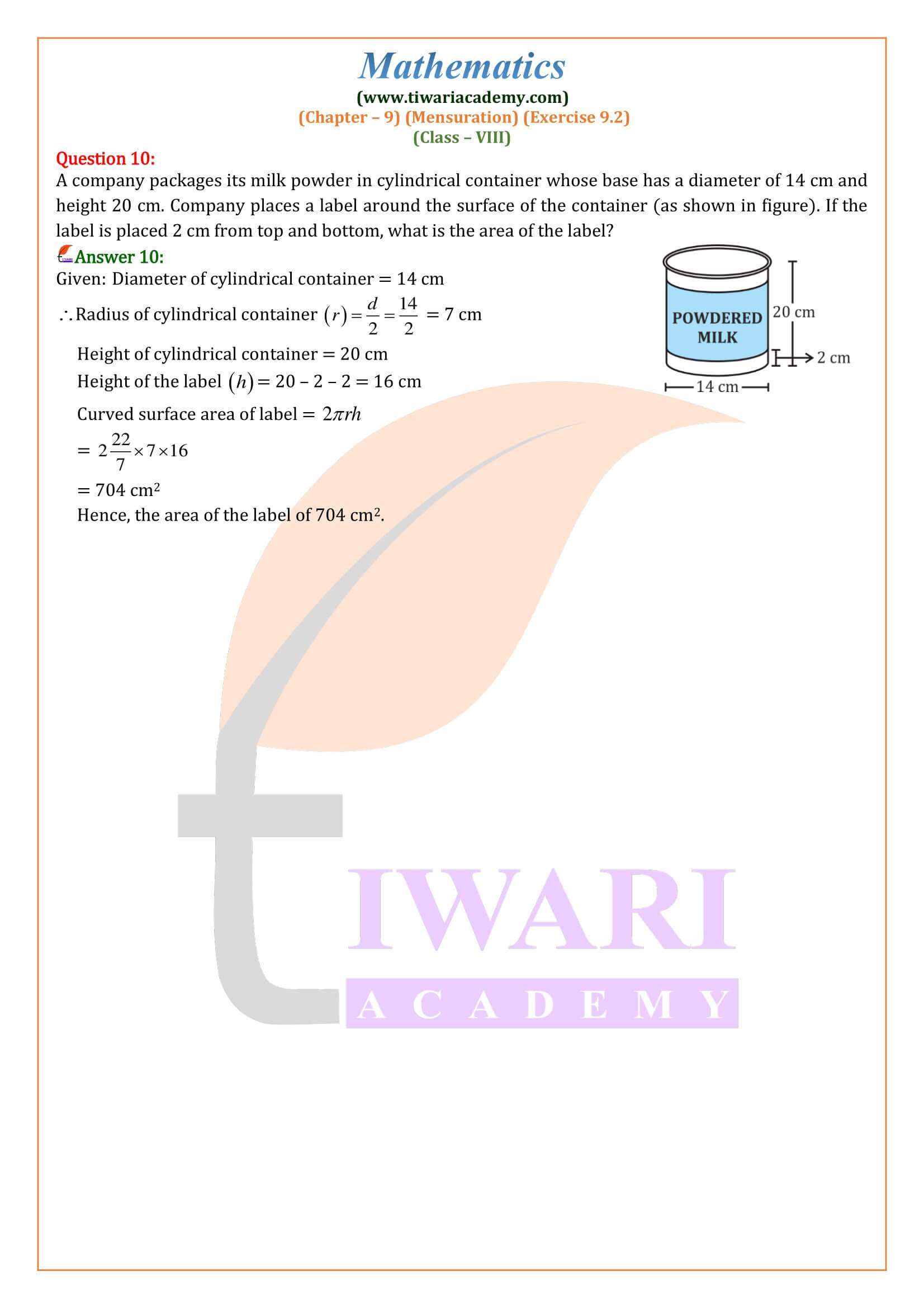NCERT Solutions for Class 8 Maths Chapter 9 Exercise 9.2 Mensuration in Hindi and English Medium updated for CBSE 2023-24 Exams. Students of grade VIII can take help during the solutions of ex. 9.2 8th mathematics revised according to new textbooks for academic year 2023-24.

• ## 8th Maths Exercise 9.2 Solution in Hindi and English Medium

 Class: 8 Mathematics Chapter: 9 Exercise: 9.2 Chapter Name: Mensuration Content: NCERT Exercise Solutions Content Type: Text and Videos Format Session: CBSE 2023-24 Medium: Hindi and English Medium

### Class 8 Maths Chapter 9 Exercise 9.2 Solution

Class VIII Mathematics Ex. 9.2 of Mensuration in PDF file format as well as online format for CBSE session 2023-24. Know here the comparison of volumes of one solid with others. Learn here how to find the areas and volumes of cube and cuboid, how it is related to cylinder or sphere. If someone has doubt to understand the NCERT textbook solutions, please try the videos solution particularly explanation video.

#### Cuboid

A solid bounded by six rectangular plane faces is called a cuboid. A matchbox, a chalkbox, a tea packet, a brick, a tile, a book, etc., are all examples of a cuboid. A cuboid has 6 rectangular faces, 12 edges and 8 vertices. Any face of a cuboid may be called its base. The four faces which meet the base are called the lateral faces of the cuboid.

#### Cube

A cuboid whose length, breadth and height are all equal is called a cube. Ice cubes, sugar cubes, dice, etc., are all examples of a cube. Each edge of a cube is called its side.

##### Volume of a Solid

The space occupied by a solid body is called its volume. The standard unit of volume is cubic centimetre, written as cubic cm or cm³.
The volume of a cube of side 1 cm is 1 cm³.
The other units of volume are mm³, dm³, m³, litre, etc.

##### Conversion of units
Length units Volume units
1 cm = 10 mm 1 cm³ = (10 x 10 x 10) mm³ = 1000 mm³ = 1 ml
dm = 10 cm 1 dm³ = (10 x 10 x 10) cm³ = 1000 cm³ = 1 l
1 m = 10 dm 1 m³ = (10 x 10 x 10) dm³ = 1000 dm³ = 1000 l
1 m = 100 cm 1 m³ = (100 x 100 x 100) cm³ = 10 cm³
###### Formulae for Volume and Surface Area of Cuboid and Cube

CUBOID

• (i) Volume of a cuboid = (length x breadth x height) = (l x b x h) cubic units
• (ii) Diagonal of a cuboid = √(l² + b² + h²) units
• (iii) Total surface area of a cuboid = 2 (lb + bh + lh) sq. units
• (iv) Lateral surface area of a cuboid = [2 (l + b) x h)] sq. units

Find the volume, the total surface area and the lateral surface area of a cuboid which is 8 m long, 6m broad and 3.5, high.
Volume of the cuboid = (l x b x h) cubic units
= (8 x 6 x 3.5) m³ = 168 m³
Total surface area of the cuboid = 2 (lb + bh + lh) sq. units
= 2 (8 x 6 + 6 x 3.5 + 8 x 3.5) m²
= 194 m²
Lateral surface area of the cuboid = {2 (l + b) x h} m²
= {2 (8 + 6) x 3.5} m²
= 98 m²

CUBE
(i) Volume of a cube = (edge)³ = a³ cubic units
(ii) Diagonal of a cube = 3a² units
(iii) Total surface area of a cube = (6a²) sq. units
(iv) Lateral surface area of a cube = (4a²) sq. units

Find the total surface area of the cube whose volume is 343 cm. Let the length of each edge of the cube be a cm.
Then, its volume = (a³) cm³
So, a³ = 343 = 7 x 7 x 7
a = 7 cm
So the length of each edge of the cube = 7 cm
Total surface area of the cube = (6a²) sq. units
= (6 x 7 x 7) cm² = 294 cm²

The volume of a reservoir is 180 m. Water is poured into it at the rate of 60 liters per minute. How many hours will it take to fill the reservoir?
Volume of the reservoir = 108 m³
= (108 x 1000) liters (Because 1 m³ = 1000 L)
Time taken to fill the reservoir
t = (volume of the reservoir in liters/ rate of flow in liters per min)
t = (108 x 1000)/60 min. = 1800 min. =1800/60 hrs. = 30 hrs.

How do you find the volume of a cube and cuboid?
Volume of cuboid = length × breadth × height.
Note: In a cuboid, when the length, breadth and height are of different units, convert them to a same unit and then solve.

What is difference between cuboid and rectangular prism?
A cuboid has a square cross-sectional area and a length, that is possibly different from the side of the cross-section. It has 8 vertices, 12 sides, 6 faces. A rectangular prism has a rectangular cross-section. It may not stand vertical, if you make it stand on the cross sectional base.

What’s the difference between a square and a cube?
The basic difference between a cube and a square is, a cube is a 3D figure (having 3 dimensions) i.e. length, breadth and height while a square has only 2 dimensions i.e. length and breadth. The sides (faces) of a cube are squares. The edges are straight lines. The corners (vertices) are at right angles.
What are the similarities between cube and cuboid?
Cube and Cuboid Similarities
(i) A cube and cuboid have six faces.
(ii) They both have 12 edges.
(iii) Cube and cuboid have eight vertices.

### What are the shapes and concepts are covered in Exercise 9.2 of chapter 9 class 8 Maths NCERT?

In exercise 9.2 of chapter 9 Mensuration from class 8 Mathematics students can learn various concepts that includes:
Measuring the area and perimeter of more than 2 dimension geometric shapes, such as cuboids, cubes, pyramids, circular cones, and right circular cylinders.
Solving problems involving the perimeter and area of simple figures.
Using formulas to find the area and volume of these shapes.
Students will also learn about the different types of units used for measuring length, and area, and how to convert between different units.

### What are the important questions of exercise 9.2 of 8th Mathematics NCERT textbook?

Question number 2 in which you are required to find the surface area of cuboids will help you to find the area of the cuboids along with the other measurements.
Questions number 6 and 10 are based on the right-angled cylinder where you will be asked to measure the area it will cover in one rotation. This means you will be asked to use the formulae of lateral surface area.
Additionally, you can solve the examples numbers 5, and 7 these will show you the step-by-step method to solve such questions and cover the concepts of exercise 9.2 from chapter 9 of class 8 Mathematics Textbook.

### How many questions are there in exercise 9.2 of class 8 Maths?

There are 10 questions in exercise 9.2 of chapter 9 Mensuration from the class 8 Mathematics NCERT book. These questions use different cases where you will use the concepts that you learned in Mensuration. These include the solving problems involving the surface area of cubes, cuboids, right-angled cylinders, and lateral surface areas.Last Edited: April 17, 2023